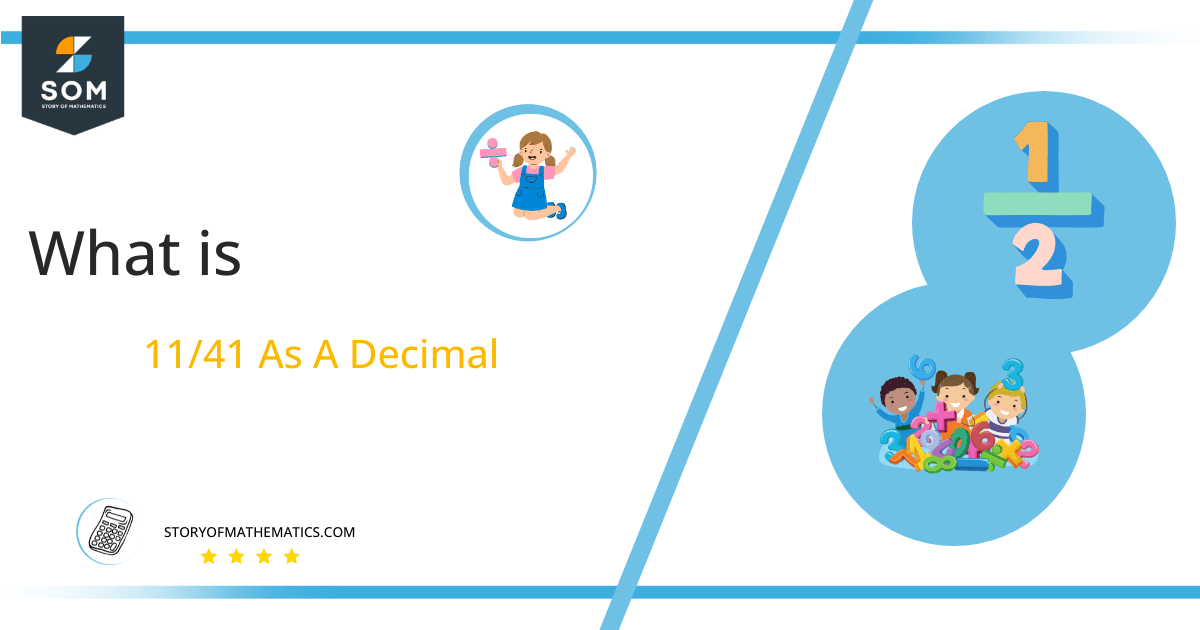# What Is 11/41 as a Decimal + Solution With Free Steps

The fraction 11/41 as a decimal is equal to 0.268.

A long division is a process used to find the decimal value of a division operation between two numbers. It has a divisor and a dividend value. The dividend is multiplied by 10 and subtracted by the closest multiple of the divisor. The remainder value is again multiplied by 10 and the process is repeated. The quotient value acquired is the resulting answer.Here, we are more interested in the division types that result in a Decimal value, as this can be expressed as a Fraction. We see fractions as a way of showing two numbers having the operation of Division between them that result in a value that lies between two Integers.

Now, we introduce the method used to solve said fraction to decimal conversion, called Long Division, which we will discuss in detail moving forward. So, let’s go through the Solution of fraction 11/41.

## Solution

First, we convert the fraction components, i.e., the numerator and the denominator, and transform them into the division constituents, i.e., the Dividend and the Divisor, respectively.

This can be done as follows:

Dividend = 11

Divisor = 41

Now, we introduce the most important quantity in our division process: the Quotient. The value represents the Solution to our division and can be expressed as having the following relationship with the Division constituents:

Quotient = Dividend $\div$ Divisor = 11 $\div$ 41

This is when we go through the Long Division solution to our problem. Given is the long division process in Figure 1:Figure 1

## 11/41 Long Division Method

We start solving a problem using the Long Division Method by first taking apart the division’s components and comparing them. As we have 11 and 41, we can see how 11 is Smaller than 41, and to solve this division, we require that 11 be Bigger than 41.

This is done by multiplying the dividend by 10 and checking whether it is bigger than the divisor or not. If so, we calculate the Multiple of the divisor closest to the dividend and subtract it from the Dividend. This produces the Remainder, which we then use as the dividend later.

Now, we begin solving for our dividend 11, which after getting multiplied by 10 becomes 110.

We take this 110 and divide it by 41; this can be done as follows:

110 $\div$ 41 $\approx$ 2

Where:

41 x 2 = 82

This will lead to the generation of a Remainder equal to 110 – 82 = 28. Now this means we have to repeat the process by Converting the 28 into 280 and solving for that:

280 $\div$ 41 $\approx$ 6

Where:

41 x 6 = 246

This, therefore, produces another Remainder which is equal to 280 – 246 = 34. Now we must solve this problem to Third Decimal Place for accuracy, so we repeat the process with dividend 340.

340 $\div$ 41 $\approx$ 8

Where:

41 x 8 = 328

Finally, we have a Quotient generated after combining the three pieces of it as 0.268, with a Remainder equal to 12.Images/mathematical drawings are created with GeoGebra.